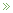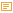• ## ROC Area-Under-the-Curve Explained

Posted by Konstantin 12.10.2011 16 Comments

The Receiver Operating Characteristic Area Under the Curve (ROC AUC) is a measure of classifier performance, which is widely used in machine learning. Unfortunately, the obscure way the concept is explained in most sources makes it fairly hard to grasp its intuitive meaning. The name "area under the curve" itself is ill-conceived and is utterly useless in helping the intution. The aim of this post is to aid those struggling with the concept, and also present a simple and intuitive interpretation of the ROC AUC metric as the "average positive rank" which I, so far, have not seen stated explicitly elsewhere.

To facilitate explanation, let us consider a hypothetical classification problem: classifying chocolate bars to "very good" or "just OK" (hereby we presume a chocolate bar may not be considered "bad" by definition of chocolate). Suppose we have a sample of particular chocolate bar types, for which we have carefully assigned the proper classification, e.g.:

Item Class
Mars 0
Milka 1
Kalev 0
Lindt 1
Ferrero-Rocher 1
Laima 0
Hershey's 0

where class label "1" denotes "very good" and "0" means "just OK". Suppose we have derived a classifier which, based on some features of a chocolate bar, such as its chocolate content, packaging, country of origin, etc, attempts to assign a "goodness score" which should resemble our notion of goodness. For concreteness' sake, let us say the classifier assigned the following scores:

Item True class Assigned score
Milka 1 8
Mars 0 5
Lindt 1 3
Ferrero-Rocher 1 3
Kalev 0 1
Laima 0 -3
Hershey's 0 -5

How do we assess the goodness of such a classifier? The "ROC approach" suggests we do the following. First, we shall plot a ROC curve. To do that, we order the chocolate bars according to their assigned score (this is already done in the table above) and use this ordering to write out the vector of the class labels (the "sorted prediction vector"):

Highest rated item's true class Lowest rated item's true class
1 0 1 1 0 0 0

Obviously, the best possible classifier would order the instances so that all the ones would be on the left of all the zeroes. The particular classifier we chose here looks good, but not perfect.

Next, we use the obtained vector to plot the ROC curve. To do this plot, forget all of the "True Positive Rate" and "False Positive Rate" nonsense you might have read about in most textbooks, and follow the following simple recipe:

1. Draw a square with sides of length 1. Denote its its lower left corner as the "origin", its horizontal axis as "the negatives" and its vertical axis as "the positives". Split the negatives axis in as many parts as there are examples of class "0" in the dataset. Split the positives axis in as many parts as there are examples of class "1" in your dataset. In our case, the square will look as follows:

2. Next, take the sorted prediction vector, and draw a path, starting from the origin. For each "1" in the sorted prediction vector, the path will move one step "up", and for each "0" in the vector, the path will move one step "right" (note that if you have multiple predictions with the same score, make all the corresponding "up" and "right" moves at once by drawing the corresponding diagonal). In our case, the path goes ("up", "right", "up", "up", "right", "right", "right") and hence looks as follows:

Voilà, this is your ROC curve. Obviously, the "perfect" classifier would make all the "up" steps first and the "right" steps last, hence the ideal ROC curve looks like a perfect corner. A random classifier would mix his "up" and "right" steps randomly, and the curve would most probably follow a diagonal-ish path. The one-number summary of the "goodness" of such a path is the "area under the curve" (coloured gray here), which will be 1.0 for the ideal case, and somewhere around 0.5 for the worst case. In our case the area is 10/12 ~ 0.83.

Here comes the important question — what does this number actually mean? One good answer is given by the following probabilistic interpretation:

The area under ROC curve specifies the probability that, when we draw one positive and one negative example at random, the decision function assigns a higher value to the positive than to the negative example.

There is, however, yet another, to my mind even more intuitive, interpretation. Define the rank of a positive example as the proportion of negative examples "to the right of it" in the sorted prediction vector. For example, if the sorted prediction vector is (1, 0, 0, 0, 0), then the rank of the sole positive item there is 1.0 (all negative examples are to the right). If the sorted prediction vector is (0, 0, 1, 0, 0), the rank is 0.5 (half of negative examples is to the right), and if the vector is (0, 0, 0, 0, 1), the rank is 0. In our chocolate bar example, the ranks of the positive examples are the following:

Highest rated item's true class Lowest rated item's true class
Sorted prediction vector 1 0 1 1 0 0 0
Rank 1.0 0.75 0.75

The "rank" thus denotes where would a classifier position a given positive item within the set of negative examples, whether closer to the "left" (rank 1) or closer to the "right" (rank 0).

Now the average rank of all the positive examples seems like a reasonable metric of classifier performance, doesn't it? In fact, is exactly the ROC AUC! Now the value of 0.80 ROC score can be interpreted intuitively as follows: "the average positive example has about 20% of negative examples scored higher than it". This, so far, seems to me personally to be the "simplest" interpretation of the ROC AUC. So write it down:

ROC AUC is the average positive rank.

• ## Un-ordering Data

Posted by Konstantin 11.10.2009 2 Comments

I've recently stumbled upon a simple observation, which does not seem to be common knowledge and yet looks quite enlightening. Namely: polynomials provide an excellent way of modeling data in an order-agnostic manner.

The situations when you need to represent data in an order-agnostic way are actually fairly common.  Suppose that you are given a traditional sample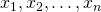and are faced with a task of devising a generic function of the sample, which could only depend on the values in the sample, but not on the ordering of these values. Alternatively, you might need to prove that a given statistic is constant with respect to all permutations of the sample. Finally, you might simply wish to have a convenient mapping for your feature vectors that would lose the ordering information, but nothing else.

The most common way of addressing this problem is sorting the sample and working with the order statistics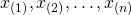instead of the original values. This is not always convenient. Firstly, the mapping of the original sample to the corresponding vector of order statistics (i.e. the sorting operation) is quite complicated to express mathematically. Secondly, the condition that the vector of order statistics is always sorted is not very pleasant to work with. A much better idea is to represent your data as a polynomial of the form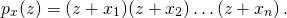This will immediately provide you with a marvellous tool: two polynomials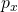andare equal if and only if their roots are equal, which means, in our case, that the samplesand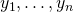are equal up to a reordering.

Now in order to actually represent the polynomial we can either directly compute its coefficients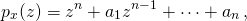or calculate its values at anydifferent points (e.g. at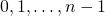) - in any case we end up with the same amount of data as we had originally (i.e.values), but the new representation is order-agnostic and has, arguably, much nicer properties than the order statistics vector.

It is not without its own problems, of course. Firstly, it requires at least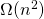time to compute. Secondly, not every polynomial will havereal-valued roots. And thirdly, the interpretation of the new "feature vector" is not necessarily intuitive or meaningful. Yet nonetheless, it's a trick to consider.

• ## What are pseudorandom numbers and what are they used for?

Posted by Swen 25.08.2009 No Comments

There seems to be a wide split of opinions between theoreticians and practitioners. Namely, some theoreticians define pseudorandomness in terms of complexity theory. As a result, the corresponding constructions are inherently slow and thus rejected by practitioners. This short blog post tries to address some common misconceptions.

Formally, a pseudorandom generator is a deterministic function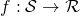which takes a (relatively short) seed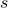and converts it into an element of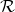. In most cases, we as computer scientists are interested in functions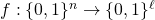. However, there are other reasonable domains, too. For instance, when performing Monte-Carlo integration in the region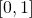, we would be interested in the functions of type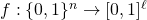.

It is important to note that any function of typeis a pseudorandom generator. However, not all of those functions are equally good for all purposes. There are two main properties that are used to discriminate between various pseudorandom functions. First, we can talk about the efficiency of a pseudorandom generator, i.e., how fast can we compute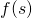. Second, we can talk about the statistical properties of the output.

Before going into the details, let us establish why anyone should use pseudorandom generators at all. There is an interesting controversy in the computer science, as well in statistics. Many algorithms assume that it is possible to use uniformly distributed numbers. For example, Monte-Carlo integration is based on the law of large numbers:whenever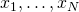are taken independently and uniformly from the range. Similarly, one can provide theoretical bounds for the randomized version of quicksort, provided that we can draw elements uniformly from a set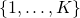. However, computers are mostly made of deterministic parts and it turns out to be really difficult to automatically collect uniformly distributed bits. A design of a device that would solve this problem is far from trivial.

The first official solution to this problem was posed in 1927 when a student of Karl Pearson published a table of random numbers. Later such tables were built by the RAND Corporation. That is, the function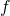was explicitly specified through a big table. Of course, such a table is useful only if it can be used as a "reliable" source of random numbers. In particular, the value of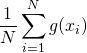should be as close to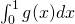as possible. Since there are infinite number of functions, we cannot actually check this condition. Instead, statisticians performed a series of tests on the table to verify that the sequence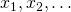looks as close to random as possible.  If we extend this concept properly, we get the modern quantification of pseudorandomness.

Formally, a functionis a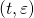-secure pseudorandom generator if for any-time algorithm:where the probabilities are taken over the uniform choices ofand. In more intuitive terms, if you replace the randomnesswithand your algorithm runs in time, then the switch generates no easily observable discrepancies.

As an illustrative example, consider a probabilistic combinatorial search algorithm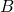that runs in timeand outputs a solution that can be verified in time. In this case, the use of a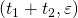-secure pseudorandom generator withininstead of pure randomness would decrease the probability offinding a solution by at most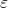. Indeed, otherwise we could construct a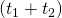-time algorithm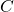that outputs 1, iffinds the solution and 0 otherwise, and use it to discern the pseudorandom generator from true randomness with success at least. This would contradict the fact that we have a true-secure pseudorandom generator.  Similar argument can be proven also for the Monte-Carlo integration algorithm. Note that parametersandcan be arbitrary real numbers. For the combinatorial search algorithm that takes 3 weeks CPU time, you might use a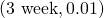-secure pseudorandom generator.

There are essentially two reasonable complaints about the pseudorandom generators. First, since obtaining random bits is hard, we have not solved the problem completely, as we must still get the seed from somewhere. This is indeed a valid problem. For instance, the standard rand() function in C is known to fail the NIST statistical test and thus you might actually observe inconsistencies when using rand() directly or generating a seed for a more complex function with rand(). The latter does not mean that rand() is not a pseudorandom generator, rather that its quality might be low for certain applications. As a complete solution, you would like to get a function. For some tasks, such as Monte-Carlo integration of certain functions the corresponding solution is known (see multidimensional integration and sparse grids).

However, we still do not know how to do it in general, i.e., how to convert randomized algorithms into deterministic ones without significant losses in their performance. The corresponding area of research is known as derandomization. Second, one might argue that we could prove directly that by replacingwith, the performance of the algorithm does not deteriorate much. However, this is not an easy task and it is usually not a valid option for usual mortals.

To summarize, whenever you use a certain functionto extend a random seed in your implementation you actually have to believe that it does not affect performance, i.e.,is a pseudorandom generator with appropriateparameter values. Whether you should use, rand() in C, or something more elaborate, depends on the application.

### Calendar

September 2023
M T W T F S S
« Oct
123
45678910
11121314151617
18192021222324
252627282930